# Plotting Options and Animations#

Demonstrate many features of the PyVista plotting API to create compelling 3D visualizations and touch on animations (10 min for talk, 10 min for exercise)

Tip

This section of the tutorial was adopted from the Plotting section of PyVista’s Example Gallery.

PyVista enables many possibilities for altering how you display 3D data, a few of our most common features include:

• Color mapping scalar values with `Matplotlib` colormaps

• Showing the edges and nodes of different mesh types

• Label points in 3D space along side your meshes

• Creating side-by-side comparisons

• Making a dataset transparent or using a scalar value to map opacity

• Adding textures/images draped over a mesh (texture mapping)

• Use sophisticated lighting techniques like smooth shading or Eye Dome Lighting

• Creating animations as GIFs or movie files

This section will overview PyVista’s `pyvista.Plotter` API and how to perform these tasks. The goal of this lesson is not to be a comprehensive overview of PyVista’s plotting API, but rather to demonstrate how it works and how you can learn to use it!

## The Basics#

PyVista’s plotting API is data-centric, where the 3D data are individually added to the scene with different display parameters in a Matplotlib-like fashion.

### Add Mesh to Plotter Object#

When plotting, users must first create a `pyvista.Plotter` instance (much like a Matplotlib figure). Then data are added to the plotter instance through the `pyvista.Plotter.add_mesh()` method. This workflow typically looks like:

```import pyvista as pv
from pyvista import examples

mesh = pv.Wavelet()

p = pv.Plotter()
p.show()
```You can customize how that mesh is displayed through the parameters of the `pyvista.Plotter.add_mesh()` method. For example, we can change the colormap via the `cmap` argument:

```p = pv.Plotter()
p.show()
```Or show the edges of the mesh with `show_edges`:

```p = pv.Plotter()
p.show()
```Or adjust the opacity to be a scalar value or linear transfer function via the `opacity` argument:

```import pyvista as pv
from pyvista import examples

p = pv.Plotter()
p.show()
```

Take a look at all of the options for add_mesh.

The `add_mesh` method can be called over and over to add different data to the same `Plotter` scene. For example, we can create many different mesh objects and plot them together:

```import pyvista as pv
from pyvista import examples

kinds = [
'tetrahedron',
'cube',
'octahedron',
'dodecahedron',
'icosahedron',
]
centers = [
(0, 1, 0),
(0, 0, 0),
(0, 2, 0),
(-1, 0, 0),
(-1, 2, 0),
]

solids = [pv.PlatonicSolid(kind, radius=0.4, center=center) for kind, center in zip(kinds, centers)]

p = pv.Plotter(window_size=[1000, 1000])
for solid in solids:
solid, color='silver', specular=1.0, specular_power=10
)
p.view_vector((5.0, 2, 3))
p.show()
```

### Subplotting#

Creating side-by-side comparisons of datasets is easy with PyVista’s subplotting API. Get started by specifying the shape of the `pyvista.Plotter` object then registering the active subplot by the `pyvista.Plotter.subplot()` method much like how you subplot with Matplotlib’s API.

```import pyvista as pv

p = pv.Plotter(shape=(1, 2))

p.subplot(0, 0)

p.subplot(0, 1)

p.show()
```

Below is an example of side-by-side comparisons of the contours and slices of a single dataset.

Tip

You can link the cameras of both views with the `pyvista.Plotter.link_views()` method

```import pyvista as pv

mesh = pv.Wavelet()
cntr = mesh.contour()
slices = mesh.slice_orthogonal()

p = pv.Plotter(shape=(1, 2))

p.subplot(0, 1)

p.view_isometric()
p.show()
```

Other custom layouts are supported by the `shape` argument as string descriptors:

• `shape="3|1"` means 3 plots on the left and 1 on the right,

• `shape="4/2"` means 4 plots on top and 2 at the bottom.

Here is an example of three plots on the right and one on the left:

```import pyvista as pv

mesh = pv.Wavelet()
cntr = mesh.contour()
slices = mesh.slice_orthogonal()
thresh = mesh.threshold(200)

p = pv.Plotter(shape="1|3")

p.subplot(1)

p.subplot(2)

p.subplot(3)

p.subplot(0)

p.view_isometric()
p.show()
```

Note

There is a comprehensive overview of subplotting in the Multi-Window Plotting Example This example details how to create more complex layouts.

## Controlling the Scene#

Tip

For a full list of methods on the `pyvista.Plotter`, please see the API documentation

### Axes and Bounds#

Axes can be added to the scene with `pyvista.Plotter.show_axes()`

```import pyvista as pv
from pyvista import examples

p = pv.Plotter()
p.show_axes()
p.show()
```

And bounds similarly with `pyvista.Plotter.show_bounds()`

Tip

See Plotting Bounds for more details.

```import pyvista as pv
from pyvista import examples

p = pv.Plotter()
p.show_axes()
p.show_bounds()
p.show()
```

## Exercises#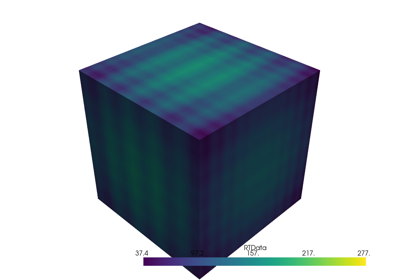Lesson Overview

Lesson Overview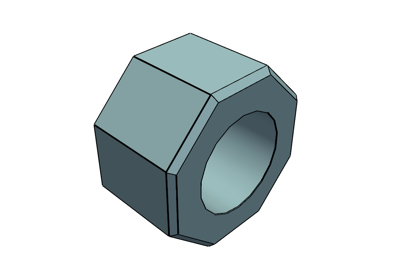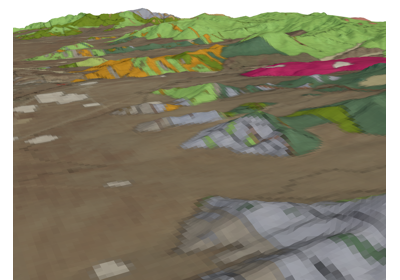Geological Map on Topography

Geological Map on Topography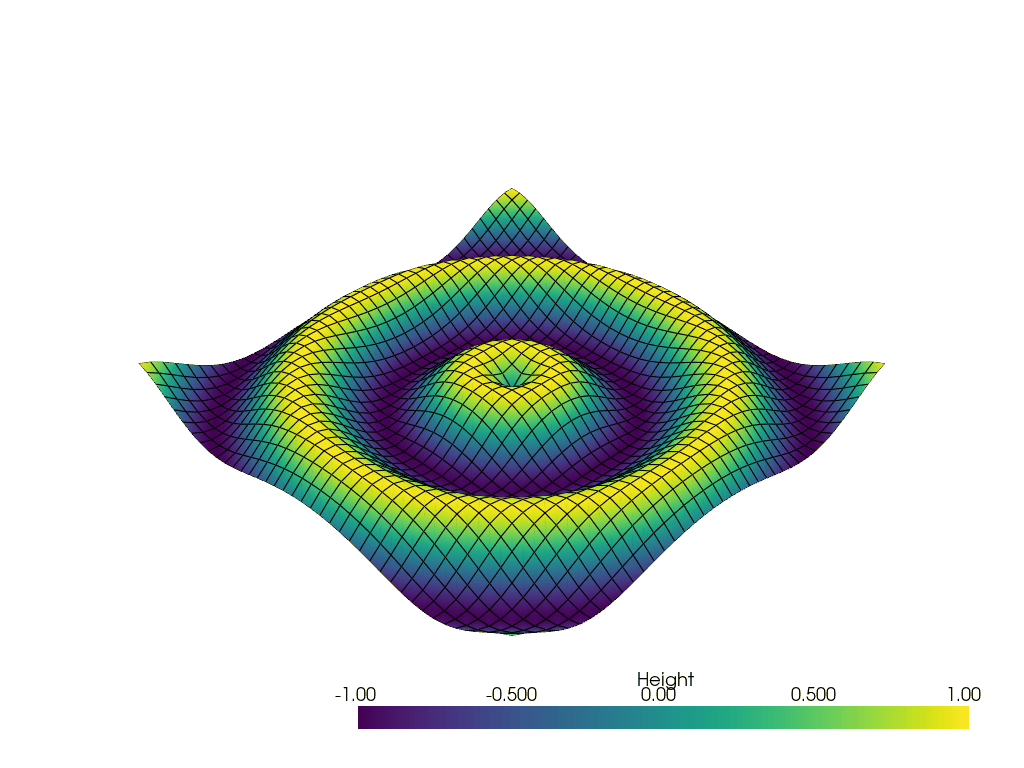Create a GIF Movie

Create a GIF Movie

### Bonus Content#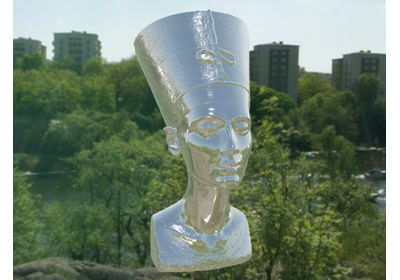Physically Based Rendering

Physically Based Rendering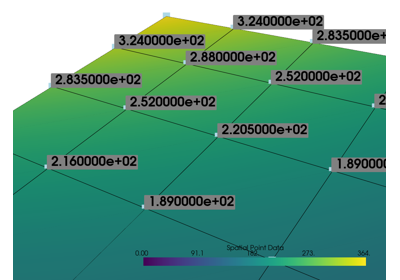Label Points

Label Points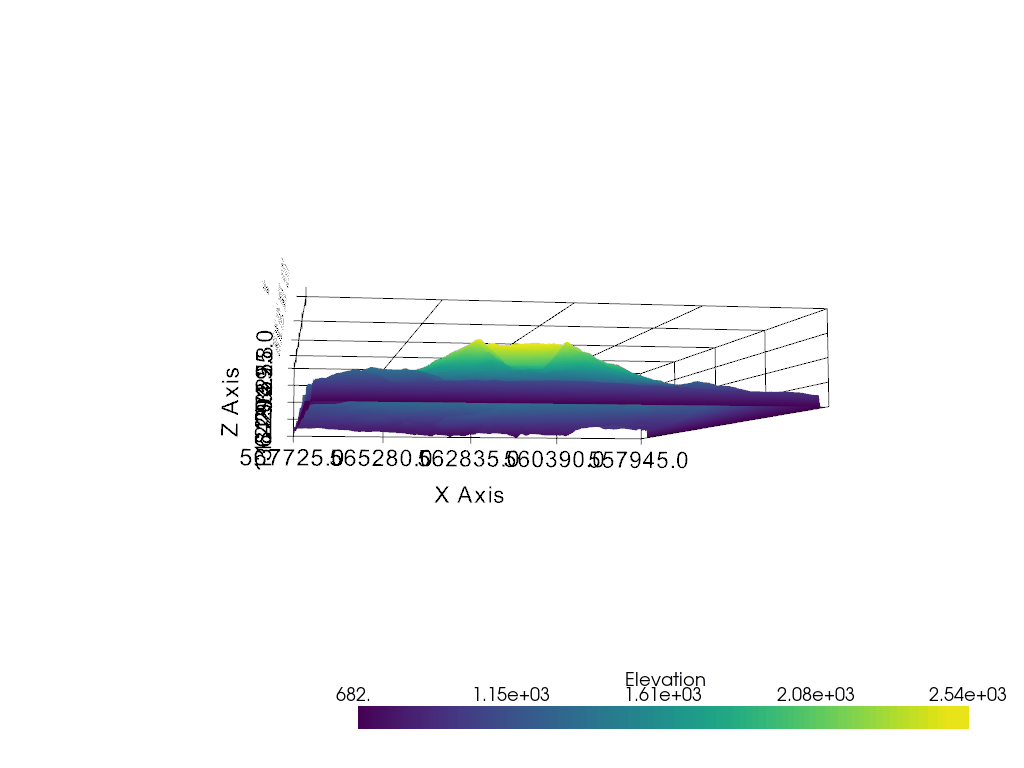Orbiting

Orbiting

### Do it yourself#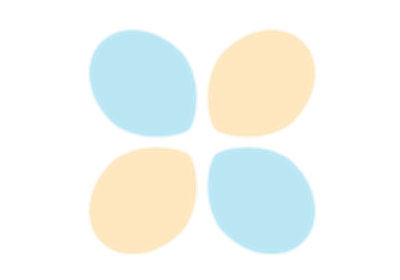Display Options

Display OptionsLighting Properties

Lighting PropertiesEye Dome Lighting

Eye Dome Lighting

### Solutions#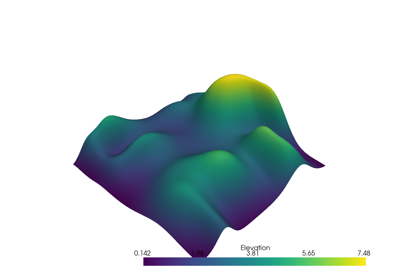Display Options

Display Options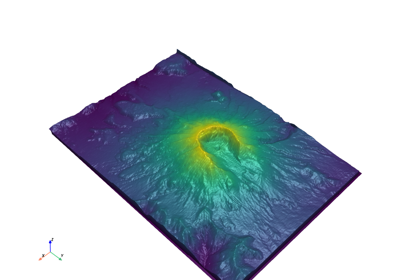Lighting Properties

Lighting Properties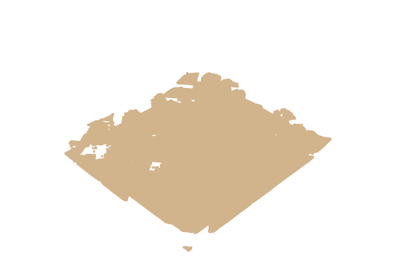Eye Dome Lighting

Eye Dome Lighting

Gallery generated by Sphinx-Gallery